# entropy

(redirected from Disorder (thermodynamics))
Also found in: Dictionary, Thesaurus, Medical, Financial.

## entropy

(ĕn`trəpē), quantity specifying the amount of disorder or randomness in a system bearing energyenergy,
in physics, the ability or capacity to do work or to produce change. Forms of energy include heat, light, sound, electricity, and chemical energy. Energy and work are measured in the same units—foot-pounds, joules, ergs, or some other, depending on the system of
or information. Originally defined in thermodynamicsthermodynamics,
branch of science concerned with the nature of heat and its conversion to mechanical, electric, and chemical energy. Historically, it grew out of efforts to construct more efficient heat engines—devices for extracting useful work from expanding hot gases.
in terms of heat and temperature, entropy indicates the degree to which a given quantity of thermal energy is available for doing useful work—the greater the entropy, the less available the energy. For example, consider a system composed of a hot body and a cold body; this system is ordered because the faster, more energetic molecules of the hot body are separated from the less energetic molecules of the cold body. If the bodies are placed in contact, heat will flow from the hot body to the cold one. This heat flow can be utilized by a heat engine (device which turns thermal energy into mechanical energy, or work), but once the two bodies have reached the same temperature, no more work can be done. Furthermore, the combined lukewarm bodies cannot unmix themselves into hot and cold parts in order to repeat the process. Although no energy has been lost by the heat transfer, the energy can no longer be used to do work. Thus the entropy of the system has increased. According to the second law of thermodynamics, during any process the change in entropy of a system and its surroundings is either zero or positive. In other words the entropy of the universe as a whole tends toward a maximum. This means that although energy cannot vanish because of the law of conservation of energy (see conservation lawsconservation laws,
in physics, basic laws that together determine which processes can or cannot occur in nature; each law maintains that the total value of the quantity governed by that law, e.g., mass or energy, remains unchanged during physical processes.
), it tends to be degraded from useful forms to useless ones. It should be noted that the second law of thermodynamics is statistical rather than exact; thus there is nothing to prevent the faster molecules from separating from the slow ones. However, such an occurrence is so improbable as to be impossible from a practical point of view. In information theoryinformation theory
or communication theory,
mathematical theory formulated principally by the American scientist Claude E. Shannon to explain aspects and problems of information and communication.
the term entropy is used to represent the sum of the predicted values of the data in a message.

## Entropy

A function first introduced in classical thermodynamics to provide a quantitative basis for the common observation that naturally occurring processes have a particular direction. Subsequently, in statistical thermodynamics, entropy was shown to be a measure of the number of microstates a system could assume. Finally, in communication theory, entropy is a measure of information. Each of these aspects will be considered in turn. Before the entropy function is introduced, it is necessary to discuss reversible processes.

#### Reversible processes

Any system under constant external conditions is observed to change in such a way as to approach a particularly simple final state called an equilibrium state. For example, two bodies initially at different temperatures are connected by a metal wire. Heat flows from the hot to the cold body until the temperatures of both bodies are the same. It is common experience that the reverse processes never occur if the systems are left to themselves; that is, heat is never observed to flow from the cold to the hot body. Max Planck classified all elementary processes into three categories: natural, unnatural, and reversible. Natural processes do occur, and proceed in a direction toward equilibrium. Unnatural processes move away from equilibrium and never occur. A reversible process is an idealized natural process that passes through a continuous sequence of equilibrium states.

#### Entropy function

The state function entropy S puts the foregoing discussion on a quantitative basis. Entropy is related to q, the heat flowing into the system from its surroundings, and to T, the absolute temperature of the system. The important properties for this discussion are:

1. dS > q/T for a natural change.

dS = q/T for a reversible change.

2. The entropy of the system S is made up of the sum of all the parts of the system so that . See Heat, Temperature

#### Nonconservation

In his study of the first law of thermodynamics, J. P. Joule caused work to be expended by rubbing metal blocks together in a large mass of water. By this and similar experiments, he established numerical relationships between heat and work. When the experiment was completed, the apparatus remained unchanged except for a slight increase in the water temperature. Work (W) had been converted into heat (Q) with 100% efficiency. Provided the process was carried out slowly, the temperature difference between the blocks and the water would be small, and heat transfer could be considered a reversible process. The entropy increase of the water at its temperature T is ΔS = Q/T = W/T. Since everything but the water is unchanged, this equation also represents the total entropy increase. The entropy has been created from the work input, and this process could be continued indefinitely, creating more and more entropy. Unlike energy, entropy is not conserved. See Conservation of energy, Thermodynamic processes

Energy is never destroyed. But in the Joule friction experiment and in heat transfer between bodies, as in any natural process, something is lost. In the Joule experiment, the energy expended in work now resides in the water bath. But if this energy is reused, less useful work is obtained than was originally put in. The original energy input has been degraded to a less useful form. The energy transferred from a high-temperature body to a lower-temperature body is also in a less useful form. If another system is used to restore this degraded energy to its original form, it is found that the restoring system has degraded the energy even more than the original system had. Thus, every process occurring in the world results in an overall increase in entropy and a corresponding degradation in energy.

#### Measure of information

The probability characteristic of entropy leads to its use in communication theory as a measure of information. The absence of information about a situation is equivalent to an uncertainty associated with the nature of the situation. This uncertainty is the entropy of the information about the particular situation.

## entropy

(en -trŏ-pee) A measure of the amount of disorder in a physical system. It never decreases in any physical interaction of a closed system.

## entropy

see SYSTEMS THEORY.

## Entropy

a concept first introduced in thermodynamics to define the measure of the irreversible dissipation of energy (seeTHERMODYNAMICS). Entropy is also extensively used in other branches of science: in statistical mechanics as a measure of the probability of the realization of some macroscopic state and in information theory as a measure of the uncertainty of some experiment or test, which may have different outcomes. These interpretations of entropy have a profound intrinsic relationship. For example, all the most important principles of statistical mechanics can be deduced on the basis of the conceptions of entropy in information theory.

The concept of entropy was introduced in thermodynamics by R. Clausius (1865), who showed that the process of the conversion of heat to work follows a general physical principle, the second law of thermodynamics (seeTHERMODYNAMICS, SECOND LAW OF). The law can be given a rigorous mathematical formulation if we introduce a specific function of state—entropy.

Thus, for a thermodynamic system undergoing a cyclic process that is quasistatic (infinitesimally slow), in which the system gradually acquires small amounts of heat δQ at corresponding absolute temperatures T, the integral of the “reduced” amount of heat δQ/T throughout the cycle is equal to zero: ∮δQ/T = 0 (the Clausius equality). Clausius derived the equation, which is equivalent to the second law of thermodynamics for equilibrium processes, by considering an arbitrary cyclic process as the sum of a very large (approaching infinity as a limit) number of elementary reversible Carnot cycles (seeCARNOT CYCLE). Mathematically, the Clausius equality is necessary and sufficient to make the expression

(1) dS = δQ/T

a total differential of the function of state S called entropy (the differential definition of entropy). The entropy difference of a system in two arbitrary states A and B (defined, for example, by the values of temperature and volume) is equal to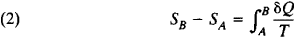(the integral definition of entropy). In this case, the integration is carried out along the path of any quasistatic process that connects states A and B, the entropy increment ΔS = SBSA being independent of the path of integration in accordance with the Clausius equality.

Thus, the second law of thermodynamics implies that there is a single-valued function of state 5 that remains constant during quasistatic adiabatic processes (δQ = 0 ). Processes in which the entropy remains constant are called isentropic. An example is adiabatic demagnetization, a process widely used to produce low temperatures (seeMAGNETIC COOLING). The change in entropy during isothermal processes is equal to the ratio between the heat transferred to the system and absolute temperature. For example, the change in entropy upon the evaporation of a liquid is equal to the ratio between the heat of vaporization and the temperature of vaporization, assuming a state of equilibrium between the liquid and its saturated vapor.

According to the first law of thermodynamics (the law of conservation of energy), δQ = dU + pdV, that is, the amount of heat transferred to the system is equal to the sum of the increment of internal energy dU and the work pdV done by the system, where p is the pressure and V is the volume of the system (seeTHERMODYNAMICS, FIRST LAW OF). With consideration of the first law of thermodynamics, the differential definition of entropy takes the form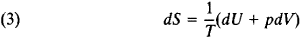which implies that when the internal energy U and the volume V are taken as the independent variables, the partial derivatives of entropy are related to absolute temperature and pressure by the expressions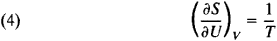and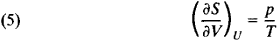These expressions are equations of state of the system: the first is the caloric equation, and the second is the heat equation (seeEQUATION OF STATE). Equation (4) is the basis for the definition of absolute temperature.

Formula (2) defines entropy only to an accuracy of an additive constant (that is, the reference point for entropy remains arbitrary). The third law of thermodynamics, or the Nernst heat theorem, makes possible the establishment of the absolute value of entropy; according to this principle, the difference ΔS of any substance approaches zero independently of external parameters as the temperature approaches absolute zero (seeTHIRD LAW OF THERMODYNAMICS). Therefore, the entropy of all substances can be taken as equal to zero at a temperature of absolute zero (M. Planck suggested this formulation of the Nernst heat theorem in 1911). On the basis of this principle, the reference point for entropy is taken as S0 = 0 when T = 0.

The importance of the concept of entropy in analyzing irreversible (nonequilibrium) processes was also first demonstrated by Clausius. For irreversible processes, the integral of the reduced heat δQ/T, over a closed path is always negative: ∮δQ/T < 0 (the Clausius inequality). This inequality is a corollary of the Carnot theorem: the efficiency of a partly or completely irreversible cyclic process is always less than the efficiency of a reversible process. The Clausius inequality implies that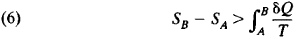and therefore the entropy of an adiabatically isolated system can only increase in irreversible processes.

Thus, entropy determines the nature of processes in an adiabatic system: the only processes that are possible are those in which entropy either remains constant (reversible processes) or increases (irreversible processes). In this connection, entropy need not increase for every body participating in the process. There is an increase in the total entropy of bodies in which the process has caused changes.

The state with maximum entropy corresponds to thermodynamic equilibrium of an adiabatic system. Entropy may have several maxima, rather than one, and in this case the system will have several equilibrium states. The equilibrium that corresponds to the greatest entropy maximum is said to be absolutely stable. The condition of maximum entropy of an adiabatic system in the equilibrium state implies an important corollary: the temperature of all parts of a system in the equilibrium state is the same.

The concept of entropy is also applicable to thermodynamically nonequilibrium states if deviations from thermodynamic equilibrium are minor, and the concept of local thermodynamic equilibrium can be introduced in small but still macroscopic volumes. Such states can be described by thermodynamic parameters, like temperature and pressure, that are weakly dependent on spatial coordinates and time, the entropy of a thermodynamically nonequilibrium state being defined as the entropy of the equilibrium state characterized by the same values of the parameters. As a whole, the entropy of a nonequilibrium system is equal to the sum of the entropies of its parts that are in local equilibrium.

The thermodynamics of nonequilibrium processes makes possible a more detailed study of the process of increasing entropy than classical thermodynamics (seeTHERMODYNAMICS, NONEQUILIBRIUM) and allows calculation of the amount of entropy formed per unit volume per unit time as a result of the system’s deviation from thermodynamic equilibrium production (seeENTROPY PRODUCTION). Entropy production is always positive and is mathematically expressed by the quadratic form of gradients of thermodynamic parameters (temperature, hydrodynamic rate, or concentrations of the components of a mixture) with kinetic coefficients (seeONSAGER THEOREM).

Statistical mechanics relates entropy to the probability that a system will be in a given macroscopic state (seeSTATISTICAL MECHANICS). Entropy here is defined in terms of the logarithm of the statistical weight Ω of the given equilibrium state:

(7) S = k ln Ω(E,N)

where k is Boltzmann’s constant and Ω(E, N) is the number of quantum-mechanical levels in a narrow energy interval ΔE close to the energy £ of a system of N particles. L. Boltzmann was the first to establish (1872) the relationship between entropy and the probability of the state of a system: the increase in entropy of a system is due to its transition from a less probable state to one that is more probable. In other words, the evolution of a closed system takes the direction of the most probable distribution of energy between individual subsystems.

In contrast to thermodynamics, statistical mechanics examines a particular class of processes—fluctuations—in which a system proceeds from a more probable state to one that is less probable, and its entropy decreases. The existence of fluctuations shows that the law of increasing entropy is satisfied on the average only for a sufficiently long time period (seeFLUCTUATION).

Entropy in statistical mechanics is closely associated with entropy in information theory, which is a measure of the uncertainty of messages of a given source (the messages are described by a set of quantities x1x2, . . ., xn, which can be, let us say, words in some language, and by corresponding probabilities p1, p2, . . ., pn of the appearance of the values of x1, x2, . . ., xn in the message). For a defined (discrete) statistical distribution of probabilities pk, entropy in information theory is the quantity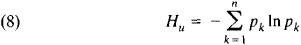with the condition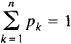The value of Hu is equal to zero if one of the pk is equal to 1 and the rest are equal to zero; that is, there is no uncertainty in the information. Entropy takes on the maximum value when the pk are all equal, and uncertainty in the information is maximum. Entropy in information theory, like entropy in thermodynamics, has the property of additivity (the entropy of several messages is equal to the sum of the entropies of the individual messages). C. E. Shannon showed that the entropy of a source of information determines the critical value of the rate of “interference-free” data transmission over a specific communication channel (Shannon’s theorem). The principal distributions of statistical mechanics can be derived from the probabilistic treatment of entropy in information theory: the canonical Gibbs distribution, which corresponds to the maximum value of informational entropy at a given average energy, and the Gibbs grand canonical ensemble, when the average energy and number of particles in the system are given.

E. Schrödinger first showed (1944) that the concept of entropy is also essential for understanding the phenomena of life. The living organism, from the viewpoint of the physicochemical processes occurring within it, can be treated as a complex open system that is in a nonequilibrium, but steady, state (seeOPEN SYSTEMS). A balance of processes leading to increased entropy and metabolic processes, which decrease entropy, is typical of organisms. However, life cannot be reduced to a simple aggregate of physicochemical processes; it also involves intricate processes of self-regulation. Therefore, the concept of entropy cannot characterize the life activity of organisms as a whole.

D. N. ZUBAREV

Entropy, in characterizing the probability that a system will be in a given state, is a measure of the state’s disorder according to (7). The change in entropy ΔS is caused both by a change in p, V, and T and by processes that proceed with p, T = const and that involve transformations of substances, including a change in their state of aggregation, dissolution, and chemical interaction.

Isothermal compression of a substance leads to a reduction of its entropy, whereas isothermal expansion and heating increase its entropy, which corresponds to equations derived from the first and second laws of thermodynamics (seeTHERMODYNAMICS):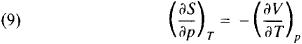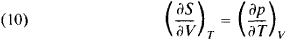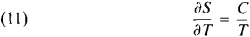Formula (11) is used for the practical determination of the absolute value of entropy at temperature T, using the Planck postulate and the values of heat capacity C and the heats and temperatures of phase transitions in the interval from zero to T°K.

In accordance with (1), entropy is measured in cal/(mole-K)— the entropy unit—or in J/(moleK). The values of entropy in the standard state are ordinarily used in calculations, most frequently at 298.15°K (25°C), that is,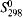; these are the entropy units used below in this’article (seeSTANDARD STATE).

Entropy increases upon the transition of a substance to a state with higher energy. AS of sublimation >ΔS of vaporization ≫ΔS of fusion >ΔS of a polymorphic transformation. For example, the entropy of water is 11.5 in the crystalline state, 16.75 in the liquid state, and 45.11 in the gaseous state.

The greater the hardness of a substance, the lower its entropy; for example the entropy of diamond (0.57 entropy unit) is half the entropy of graphite (1.37 entropy unit). Carbides, borides, and other very hard substances are characterized by low entropy.

The entropy of an amorphous solid is somewhat higher than that of a crystalline solid. An increase in the degree of dispersion of a system also leads to a certain increase in entropy.

Entropy increases with increasing complexity of a substance’s molecule; for example, the entropy is 52.6, 73.4, and 85.0 entropy units for the gases N2O, N2O3, and N2O5, respectively. The entropy of branched hydrocarbons is less than that of unbranched hydrocarbons of the same molecular mass; the entropy of a cy-cloalkane (cycloparaffin) is lower than that of its corresponding alkene.

The entropy of simple substances and compounds (for example, the chlorides ACln), as well as the changes in entropy upon melting and vaporization, are periodic functions of the ordinal number of the corresponding element. The periodicity of the change in entropy for similar chemical reactions of the type (1/n )Acryst + (1/2)Clgas = (1/n )ACn cryst practically does not appear. In the set of analog substances, such as ACl4gas’ where A is C, Si, Ge, Sn, or Pb, the entropy changes in a regufar manner. The similarity of substances (N2 and CO; CdCl2 and ZnCl2; Ag2Se and Ag2Te; BaCO3 and BaSiO3; PbWO4 and PbMoO4) is reflected in the similarity of their entropies. The discovery of a regularity in the change in entropy in a series of similar substances owing to differences in their structure and composition has made it possible to develop methods for the approximate calculation of entropy

The sign of the change in entropy ΔSC. r during a chemical reaction is determined by the sign of the change in volume of the system ΔVC. r.; however, processes like isomerization and cyclization are possible in which ΔSC. r. 0 even though ΔVC. r. ≈ 0. In accordance with the equation ΔG = ΔHTΔS, where G is Gibbs energy and H is enthalpy, the sign and absolute value of ΔSc. r. are important for judging the influence of temperature on chemical equilibrium. Spontaneous exothermal processes (ΔG < 0, ΔW < 0) that occur with a reduction of entropy (ΔS < 0) are possible. Such processes are common, in particular, in the case of dissolution (for example complexing), which is evidence of the importance of the chemical interactions between the substances that take part in these processes.

M. KH. KARAPETIANTS

### REFERENCES

Clausius, R. In Vtoroe nachalo termodinarniki. Moscow-Leningrad, 1934. Pages 71–158.
Sommerfeld, A. Termodinamika i statislicheskaia fizika. Moscow, 1955. (Translated from German.)
Mayer, J. E., and M. Goeppert-Mayer. Statisticheskaia mekhanika. Moscow, 1952. (Translated from English.)
Groot, S. de, and P. Mazur. Neravnovesnaia termodinamika. Moscow, 1964. (Translated from English.)
Zubarev, D. N. Neravnovesnaia statisticheskaia termodinamika. Moscow, 1971.
Iaglom, A. M., and I. M. Iaglom. Veroiatnost’ i informatsiia, 3rd ed. Moscow, 1973.
Brillouin, L. Nauka i teoriia informatsii. Moscow, 1959. (Translated from English.)

## entropy

[′en·trə·pē]
(communications)
A measure of the absence of information about a situation, or, equivalently, the uncertainty associated with the nature of a situation.
(mathematics)
In a mathematical context, this concept is attached to dynamical systems, transformations between measure spaces, or systems of events with probabilities; it expresses the amount of disorder inherent or produced.
(statistical mechanics)
Measure of the disorder of a system, equal to the Boltzmann constant times the natural logarithm of the number of microscopic states corresponding to the thermodynamic state of the system; this statistical-mechanical definition can be shown to be equivalent to the thermodynamic definition.
(thermodynamics)
Function of the state of a thermodynamic system whose change in any differential reversible process is equal to the heat absorbed by the system from its surroundings divided by the absolute temperature of the system. Also known as thermal charge.

## entropy

1. a thermodynamic quantity that changes in a reversible process by an amount equal to the heat absorbed or emitted divided by the thermodynamic temperature. It is measured in joules per kelvin.
2. a statistical measure of the disorder of a closed system expressed by S = klog P + c where P is the probability that a particular state of the system exists, k is the Boltzmann constant, and c is another constant

## entropy

(theory)
A measure of the disorder of a system. Systems tend to go from a state of order (low entropy) to a state of maximum disorder (high entropy).

The entropy of a system is related to the amount of information it contains. A highly ordered system can be described using fewer bits of information than a disordered one. For example, a string containing one million "0"s can be described using run-length encoding as [("0", 1000000)] whereas a string of random symbols (e.g. bits, or characters) will be much harder, if not impossible, to compress in this way.

Shannon's formula gives the entropy H(M) of a message M in bits:

H(M) = -log2 p(M)

Where p(M) is the probability of message M.

## entropy

Disorder or randomness. In data compression, it is a measure of the amount of non-redundant and non-compressible data in an object (the amount that is not similar). In encryption, it is the amount of disorder or randomness that is added. In software, it is the disorder and jumble of its logic, which occurs after the program has been modified over and over. See encryption algorithm.
Site: Follow: Share:
Open / Close